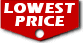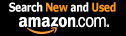# An Introduction to Stochastic Modeling / Mark A. Pinsky

List Price: \$99.95
Our Price: \$93.61For Bulk orders
Quantity

Used Book Price:
/ Mark A. Pinsky
Availability:Usually ships within 2 to 3 weeks.
Sales Rank: 688371
 ISBN-10: 0123814162ISBN-13: 9780123814166

Similar Books
 Introduction to Probability Models The Elements of Statistical Learning: Data Mining, Inference, and Prediction, Second Edition (Springer Series in Statistics) An Introduction to Mathematical Statistics and Its Applications (6th Edition) Python Data Science Handbook: Essential Tools for Working with Data An Introduction to Statistical Learning: with Applications in R (Springer Texts in Statistics) Elementary Analysis: The Theory of Calculus (Undergraduate Texts in Mathematics) Introduction to Mathematical Statistics (8th Edition) (What's New in Statistics) Complex Variables and Applications (Brown and Churchill) Generalized Linear Models (Chapman & Hall/CRC Monographs on Statistics and Applied Probability) A Second Course in Statistics: Regression Analysis (7th Edition)

Serving as the foundation for a one-semester course in stochastic processes for students familiar with elementary probability theory and calculus, the fourth edition of Introduction to Stochastic Modeling bridges the gap between basic probability and an intermediate level course in stochastic processes. The objectives of the text are to introduce students to the standard concepts and methods of stochastic modeling, to illustrate the rich diversity of applications of stochastic processes in the applied sciences, and to provide exercises in the application of simple stochastic analysis to realistic problems.

In the fourth edition, we have added two new chapters: Chapter 10 on random evolution and Chapter 11 on characteristic functions. Chapter 10, "Random Evolution," denotes a set of stochastic models, which describe continuous motion with piecewise linear sample functions. Explicit formulas are available in the simplest cases. In the general case, one has a central limit theorem, which is pursued more generally in Chapter 11, “Characteristic Functions and Their Applications.” Here the necessary tools from Fourier analysis are developed and applied when necessary. Many theorems are proved in full detail, while other proofs are sketched--in the spirit of the earlier Chapters 1-9. Complete proofs may be found by consulting the intermediate textbooks listed in the section on further reading. Instructors who have taught from the third edition may be reassured that Chapters 1-9 of the new edition are identical to the corresponding chapters of the new book.

Changes This Edition
• Realistic applications from a variety of disciplines integrated throughout the text, including more biological applications
• Plentiful, completely updated problems
• Completely updated and reorganized end-of-chapter exercise sets, 250 exercises with answers
• New chapters of stochastic differential equations and Brownian motion and related processes
• Additional sections on Martingale and Poisson process

Read a sample chapter from Introduction to Stochastic Modeling.

Now you can buy Books online in USA,UK, India and more than 100 countries.
*Terms and Conditions apply
Disclaimer: All product data on this page belongs to.
No guarantees are made as to accuracy of prices and information.